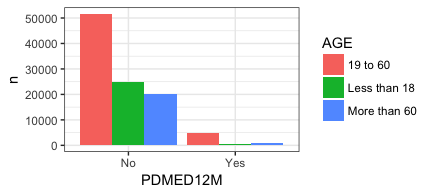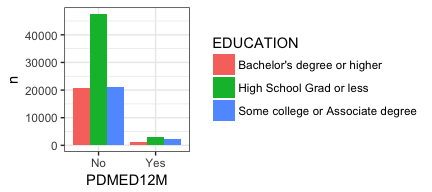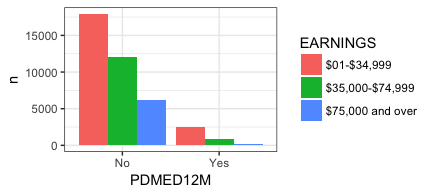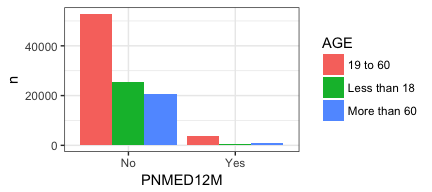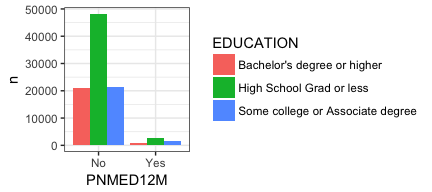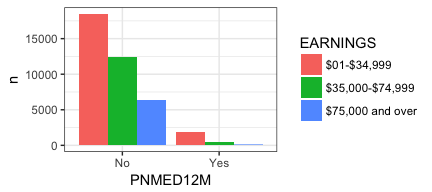In my last two posts I have been writing about the task of using R to “drive” MS Excel. The first post focused on just the basic mechanics of getting my colleague what she needed. The second post picked up with some ugly inefficient code and made it better using `lapply` and a `for loop`, just good old fashioned automation (the thing that computers excel at). Today I’ll take it another step and show how to produce the same graphs in `R` using `ggplot2` as well as how to write some simple functions to make your programming life easier.

## Background and catch-up

My colleague wanted to be able to do some simple analysis around health care using the Centers for Disease Control and Prevention, National Center for Health Statistics, National Health Interview Survey. They wanted a series of cross tabulated sets of summary data for variable pairings (for example whether or not the respondent had a formal health care provider by region of the country). They wanted one Excel “workbook” with 12 worksheets, each one of which was the summary of counts for a pair of variables. From there they could use Excel’s native plotting tools to make the graphs they needed.

You can review everything that happened in the first post, as well as the second (which I strongly recommend), or you can start on this page. To join us in progress make sure you load the right libraries and grab the dataset we wound up with from our earlier work – it’s called `OfInterest`. it represents the variables of interest for us after winnowing down a very large dataset we got from the CDC.

``````knitr::opts_chunk\$set(echo = TRUE, warning = FALSE)
library(dplyr)``````
``````##
## Attaching package: 'dplyr'

## The following objects are masked from 'package:stats':
##
##     filter, lag

## The following objects are masked from 'package:base':
##
##     intersect, setdiff, setequal, union``````
``````library(ggplot2)
theme_set(theme_bw()) # set theme to my personal preference
# install.packages("openxlsx")
require(openxlsx)``````
``## Loading required package: openxlsx``
``````OfInterest <- read.csv("ofinterest.csv")
str(OfInterest)``````
``````## 'data.frame':    103789 obs. of  9 variables:
##  \$ AGE      : Factor w/ 3 levels "19 to 60","Less than 18",..: 1 1 1 2 2 1 1 1 3 1 ...
##  \$ REGION   : Factor w/ 4 levels "Midwest","Northeast",..: 3 4 4 4 4 4 3 3 4 3 ...
##  \$ SEX      : Factor w/ 2 levels "Female","Male": 2 1 2 2 1 1 1 2 2 2 ...
##  \$ EDUCATION: Factor w/ 3 levels "Bachelor's degree or higher",..: 3 2 1 2 NA 1 2 2 1 3 ...
##  \$ EARNINGS : Factor w/ 3 levels "\$01-\$34,999",..: 1 2 2 NA NA 3 1 NA 2 3 ...
##  \$ PDMED12M : Factor w/ 2 levels "No","Yes": 1 2 1 1 1 1 1 1 1 1 ...
##  \$ PNMED12M : Factor w/ 2 levels "No","Yes": 1 1 1 1 1 1 1 1 1 1 ...
##  \$ NOTCOV   : Factor w/ 2 levels "Covered","Not covered": 1 1 1 1 1 1 1 1 1 1 ...
##  \$ MEDBILL  : Factor w/ 2 levels "No","Yes": 1 2 2 2 2 1 1 1 1 1 ...``````

## Where we left off

Yesterday we left off accomplishing this workflow:

1. Create a new empty workbook object `wb <- createWorkbook()` once
2. Invent a name for the tab or worksheet inside the workbook `NameofSheet` 12 times
3. Make a `table` for a pair of variables like `TheData` 12 times
4. Add a worksheet (tab) into the workbook `addWorksheet` 12 times
5. Write the table we made onto the worksheet with `writeData` 12 times
6. Save the workbook with the 12 sheets in it once
``````# put our variables into two lists
depvars <- list(Coverage = OfInterest\$NOTCOV, ProbPay = OfInterest\$MEDBILL, CareDelay = OfInterest\$PDMED12M, NeedNotGet = OfInterest\$PNMED12M)
indvars <- list(Education = OfInterest\$EDUCATION, Earnings = OfInterest\$EARNINGS, Age = OfInterest\$AGE)
# Use lapply to make a list that contains the tables
TablesList <- lapply(depvars, function (x) lapply(indvars, function (y) table(y,x)))
## Create a new empty workbook
wb <- createWorkbook()
## nested for loop
for (j in seq_along(TablesList)) { #top list with depvars
for (i in seq_along(TablesList[[j]])) { #for each depvar walk the indvars
TheData <- TablesList[[j]][[i]]
NameofSheet <- paste0(names(TablesList[j]), "By", names(TablesList[[j]][i]))
addWorksheet(wb = wb, sheetName = NameofSheet)
writeData(wb = wb, sheet = NameofSheet, x = TheData, borders = "n")
}
}
## Save our new workbook
saveWorkbook(wb, "newversion.xlsx", overwrite = TRUE) ## save to working directory``````

At this juncture my colleague is happy. She has her workbook nicely organized and she can do what she needs to do in MS Excel. I’m sure that included making some graphs as well. If you’re interested the `openxlsx` package even includes facilities for inserting ggplot graphics onto the work sheets.

## Better plotting through R

One thing I wanted to explore was just automating the process of plotting the data in `R` as much as I could. Basic plotting was a breeze, no need to create special lists of tables, just pipe `%>%` things straight from the dataframe into ggplot. Here are at least three possible bar or column plots of `EDUCATION` and `NOTCOV` with very little effort.

``````### with dplyr and ggplot
OfInterest %>%
filter(!is.na(EDUCATION), !is.na(NOTCOV))  %>%
group_by(EDUCATION,NOTCOV) %>%
count() %>%
ggplot(aes(fill=EDUCATION, y=n, x=NOTCOV)) +
geom_bar(position="dodge", stat="identity")``````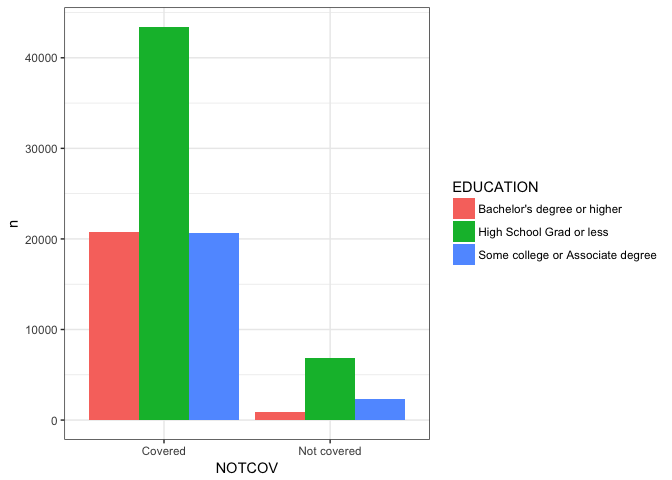``````OfInterest %>%
filter(!is.na(EDUCATION), !is.na(NOTCOV))  %>%
group_by(EDUCATION,NOTCOV) %>%
count() %>%
ggplot(aes(fill=EDUCATION, y=n, x=NOTCOV)) +
geom_bar(stat="identity")``````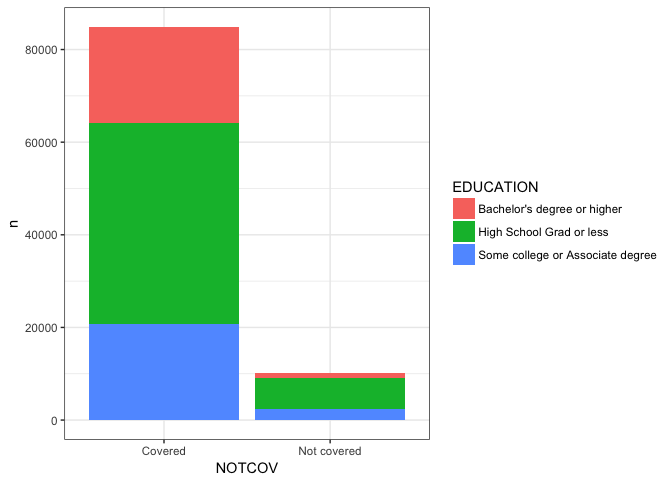``````OfInterest %>%
filter(!is.na(EDUCATION), !is.na(NOTCOV))  %>%
group_by(EDUCATION,NOTCOV) %>%
count() %>%
ggplot(aes(fill=EDUCATION, y=n, x=NOTCOV)) +
geom_bar(stat="identity", position="fill")``````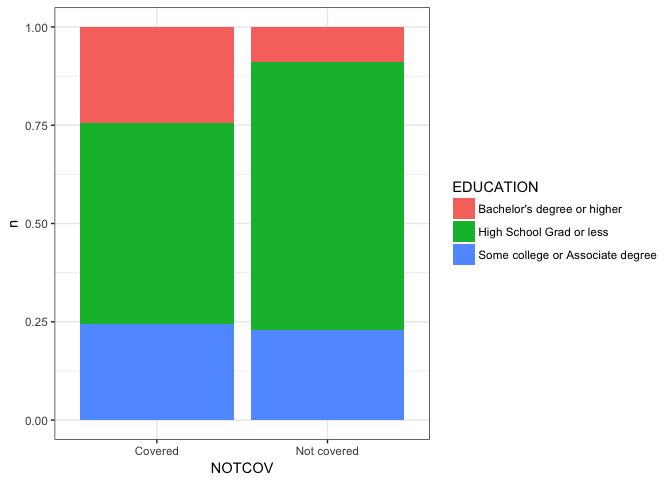Note that with our original choice of using the `table` command to create the cross tabulation that the `NA`s were silently discarded. With `dplyr` if we don’t `filter` them out we will see them plotted which may or may not be what you want substantively!

From this point forward I’m going to print the plots in a smaller size. I’m doing that via RMarkdown and it won’t happen automatically for you.

Here’s the graph with the NA’s left in place.

``````OfInterest %>%
#  filter(!is.na(EDUCATION), !is.na(NOTCOV))  %>%
group_by(EDUCATION,NOTCOV) %>%
count() %>%
ggplot(aes(fill=EDUCATION, y=n, x=NOTCOV)) +
geom_bar(stat="identity", position="fill")``````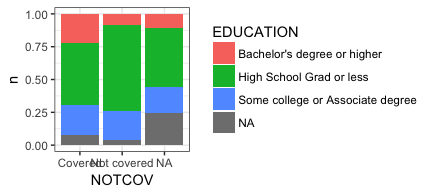I’ll leave it up to you, the reader, to decide which graph communicates any points you want to make about the data. I also acknowledge that at this point I’ve done a poor job of labeling the plot properly. We’ll come back to that later. For now I want you to focus on a couple of areas that are ripe for automation. One is improving our ability to pass multiple variables and get multiple plots. Let’s avoid cutting and pasting the code repeatedly shall we? We could also look at letting our user choose which plot type they prefer.

Now, I happen to love using `dplyr`, it is so elegant, and the syntax, plus piping, is just a joy to work with. But the downside is that it was originally designed to be used at the command prompt interactively. It makes heavy use of non standard evaluation `NSE` which makes it tricky to program functions with. Not impossible, but tricky. Hadley Wickham has written about it extensively and the Stack Overflow is full of questions about it, so I’m not sure I’m the person to explain it. But I can show a practical example of how to use it. And if you’re like me that is sometimes very helpful.

You would think (if you knew even a little bit about functions in `R`) that all we would have to do is take our code snippet from above and do some substitution… After all it’s just simple substitution right? Trust me it won’t work. Nor will any amount of traditional quoting, e.g. single quotes, double quotes, etc..

``````#### THIS WON'T WORK ####
PlotMe <- function(dataframe,x,y){
dataframe %>%
filter(!is.na(x), !is.na(y))  %>%
group_by(x,y) %>%
count() %>%
ggplot(aes(fill=x, y=n, x=y)) +
geom_bar(position="dodge", stat="identity") ->p
plot(p)
}
PlotMe(OfInterest,EDUCATION,NOTCOV)
#### THIS WON'T WORK ####``````

If you read all those references above (and your head doesn’t explode) you’ll learn how it can be done. Actually the references are very good and you will learn, I’m just here to give you a very practical example using a real world scenario.

``````PlotMe <- function(dataframe,x,y){
aaa <- enquo(x)
bbb <- enquo(y)
dataframe %>%
filter(!is.na(!! aaa), !is.na(!! bbb))  %>%
group_by(!! aaa,!! bbb) %>%
count() %>%
ggplot(aes_(fill=aaa, y=~n, x=bbb)) +
geom_bar(position="dodge", stat="identity") ->p
plot(p) # not strictly necessary
}
PlotMe(OfInterest,EDUCATION,NOTCOV)``````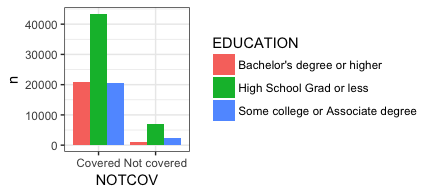## “Banging” out an NSE solution (pun intended)

If you compare the two functions you are immediately struck by a few extra lines of code that include `enquo`, a plethora of exclamation marks (a.k.a. “bang”) in the `dplyr` section and some underscores and a tilde “~” in the `ggplot` section. Let’s address them in order.

When we call the `PlotMe` function `PlotMe(OfInterest,EDUCATION,NOTCOV)` we are passing it the name of our dataset `OfInterest` and two bare variables (`EDUCATION,NOTCOV`) N.B. it wouldn’t matter if they were quoted we’d still have to do something. The problem is that `R` can’t precisely understand what we want. It’s not 100% certain that `EDUCATION` & `NOTCOV` are variables contained in `OfInterest` they could be a lot of different things. By using `enquo` initially to sort of wrap them up and then `!!` within our `dplyr` commands we can make it clear. Let’s create a new function that isolates just the `dplyr` portions of our code and ensure we’re getting a sensical answer.

``````JustDplyr <- function(dataframe,x,y){
aaa <- enquo(x)
bbb <- enquo(y)
dataframe %>%
filter(!is.na(!! aaa), !is.na(!! bbb))  %>%
group_by(!! aaa,!! bbb) %>%
count() -> JustAnExample
return(as.data.frame(JustAnExample))
}
JustDplyr(OfInterest,EDUCATION,NOTCOV)``````
``````##                          EDUCATION      NOTCOV     n
## 1      Bachelor's degree or higher     Covered 20802
## 2      Bachelor's degree or higher Not covered   895
## 3         High School Grad or less     Covered 43404
## 4         High School Grad or less Not covered  6846
## 5 Some college or Associate degree     Covered 20667
## 6 Some college or Associate degree Not covered  2313``````

Good. That’s what we want to see. Without the enquoting and the banging we’ll get consistent symptoms that it doesn’t know what `EDUCATION` & `NOTCOV` are.

`ggplot` like `dplyr` uses `NSE` by default to understand the data you are passing it in the `aes` section of the function (see ?ggplot2::aes_). That makes our life much easier for interactive work but necessitates `aes_` in this case so we can weave it into our function. `aaa` and `bbb` are already enquoted and using `aes_` ensures that `ggplot` accounts for that. `~n` is the syntax for telling `aes_` that it should find a variable called `n` inside the dataframe it is being passed from `dplyr` rather than looking for some other object named `n`.

``````#### this is just a snippet and won't run on it's own
ggplot(aes_(fill=aaa, y=~n, x=bbb)) +
geom_bar(position="dodge", stat="identity") ->p``````

A friendly reminder that as my tagline indicates I don’t consider myself a “programmer”. I love analyzing data and I love `R` but I approach programming slowly and cautiously. What I’m about to explain as my method will likely seem quaint and even antiquated to some but has the advantage of being very methodical and very practical. There are lots of places on the web to read about this stuff, I’m simply making the case I hope mine is slow and enough and methodical enough for a beginner.

We have a nice working function called `PlotMe`. We’ve proved up above that it works and having it means that if we change something inside the function (like add a title to our plot) it will apply itself every time.

We now face a similiar problem to the one we had the other day. We want to take our really cool new function and apply it to lots of variables, not constantly specify the pair we want. Let’s use the same tactic we did then and make two lists of which variables we want. This time I am deliberately choosing to make both lists shorter. That’s only because we’re going to draw plots eventually and they take up a lot of room on the screen.

The other key thing to notice compared to my earlier post is the use of `as.name`. Since we wrote our function to accept “bare variable names” we have to make sure to put bare variables names into the list. Last time we used `OfInterest\$NOTCOV` for example and `R` knew exactly what that was. If you try `list(NOTCOV, MEDBILL)` you will fail with an error message of `Error: object 'NOTCOV' not found`. If you try `depvars <- list("NOTCOV", "MEDBILL")` it won’t fail it will do worse and give you a plot that doesn’t make sense because it was expecting a bare variable name.

``````PlotMe <- function(dataframe,x,y){
aaa <- enquo(x)
bbb <- enquo(y)
dataframe %>%
filter(!is.na(!! aaa), !is.na(!! bbb))  %>%
group_by(!! aaa,!! bbb) %>%
count() %>%
ggplot(aes_(fill=aaa, y=~n, x=bbb)) +
geom_bar(position="dodge", stat="identity") ->p
#   plot(p) # not strictly necessary
}
depvars <- list(as.name("NOTCOV"), as.name("MEDBILL"))
indvars <- list(as.name("EARNINGS"), as.name("AGE"))
lapply (indvars, PlotMe, dataframe=OfInterest, y =NOTCOV)``````
``## []``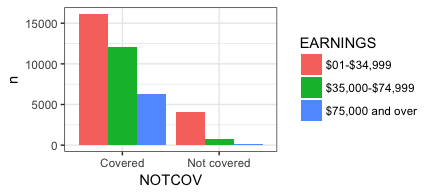``````##
## []``````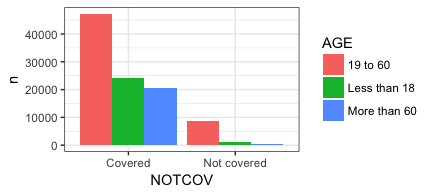``lapply (depvars, PlotMe, dataframe=OfInterest, x =EDUCATION)``
``## []````````##
## []``````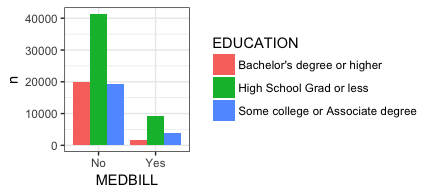``#lapply(depvars, function (x) lapply(indvars, function (y) PlotMe(dataframe=OfInterest,y=y,x=x)))``

Perfect! Just what we were looking for. We get back 4 plots. Progress! No surprise it works the other way around as well. We can hold the second part of the table command constant and just vary the independent variable via `indvars`!

What we need, of course, is both of those things. A “nested” set of calls to `lapply` to walk through both lists and give us all the tables not a subset. So I tried to do what I had earlier and nest one `lapply` inside another. For the astute among you you will notice I have that line commented out. I tried to call `lapply` and tell it to run `lapply`! The second `lapply` should in turn call `Plotme` and all we have to do is to keep our x’s and y’s correct! Miserable failure!

### Learning from failure

I did quite a few variations with no success. Perhaps one day I’ll figure it out or one of you readers will point out the error of my ways. But undaunted I sat back and tried to figure a way to snatch success from this setback. The way I saw it I had at least two problems. One, the fact that the nested `lapply` wasn’t working but also that my process was just tedious. Building lists of variable names (quoted or bare) gets tedious. It’s a lot of typing and prone to errors. Perhaps I’d have more luck if I used the fact that each of the columns in a dataframe is also numbered… `OfInterest[,1]` corresponds to `AGE`. Maybe a more efficient way of approaching this problem was to take the dependent variable column numbers and cross them with the independent variable column numbers `OfInterest[,c(1,3:4,7)]` looked like a lot less typing than expressing `AGE SEX EDUCATION PNMED12M`. So the first two lines of code below put the right variables in `xwhich` and `ywhich` and are easy to verify!

``head(OfInterest[,c(6:9)])``
``````##   PDMED12M PNMED12M  NOTCOV MEDBILL
## 1       No       No Covered      No
## 2      Yes       No Covered     Yes
## 3       No       No Covered     Yes
## 4       No       No Covered     Yes
## 5       No       No Covered     Yes
## 6       No       No Covered      No``````
``````xwhich <- c(6:9)
``````##            AGE                        EDUCATION         EARNINGS
## 1     19 to 60 Some college or Associate degree      \$01-\$34,999
## 2     19 to 60         High School Grad or less  \$35,000-\$74,999
## 3     19 to 60      Bachelor's degree or higher  \$35,000-\$74,999
## 4 Less than 18         High School Grad or less             <NA>
## 5 Less than 18                             <NA>             <NA>
## 6     19 to 60      Bachelor's degree or higher \$75,000 and over``````
``ywhich <- c(1,4,5)``

Now we have two vectors. Each of them is a set of integers for the columns that we are interested in. Our `PlotMe` function is actually expecting a bare variable name but we can produce that by using the `colnames` function `colnames(OfInterest[xwhich[]])` gives us the name of the column in `OfIinterest` that corresponds to the first element of our `xwhich` vector.

``````# First element of the vector (6)
xwhich[]``````
``##  6``
``````# The name of the column in OfInterest which corresponds to 6 quoted "PDMED12M"
colnames(OfInterest[xwhich[]])``````
``##  "PDMED12M"``
``````# The name of the column in OfInterest which corresponds to 6 bare PDMED12M
as.name(colnames(OfInterest[xwhich[]]))``````
``## PDMED12M``

Okay now we know how to move between column number and column name in two ways. That still doesn’t solve our problem with `lapply`. But `lapply` is part of a family of functions and it turns out that one of its siblings is `mapply`. When we look at the documentation for `?mapply` we see that it takes two or more lists and applies them to a function. So rather than trying to nest our call we could conceivably pass our `PlotMe` function two lists. One that contains our list of dependent variables in the right order and the other list our independent variables. First, what we need to do is “cross” them so that we get two lists of 12. Each of the lists will repeat itself as needed. We can use a `for loop` to build the lists. So our steps are:

1. Initialize an empty list for both our independent and dependent variables.
2. Create a counter so the lists stay aligned.
3. Use the `for loop` with the `as.name(colnames(OfInterest[xwhich[[j]]]))` type call to put things in our list where they belong.

To make things easy to follow I have added a `cat` statement that will print 12 times as we do this.

``````indvars<-list() # create empty list to add to
depvars<-list() # create empty list to add to
totalcombos <- 1 # keep track of where we are
for (j in seq_along(xwhich)) {
for (k in seq_along(ywhich)) {
depvars[[totalcombos]] <- as.name(colnames(OfInterest[xwhich[[j]]]))
indvars[[totalcombos]] <- as.name(colnames(OfInterest[ywhich[[k]]]))
cat("iteration #", totalcombos,
" xwhich=", xwhich[[j]], " depvars = ", as.name(colnames(OfInterest[xwhich[[j]]])),
" ywhich=", ywhich[[k]], " indvars = ", as.name(colnames(OfInterest[ywhich[[k]]])),
"\n", sep = "")
totalcombos <- totalcombos +1
}
}``````
``````## iteration #1 xwhich=6 depvars = PDMED12M ywhich=1 indvars = AGE
## iteration #2 xwhich=6 depvars = PDMED12M ywhich=4 indvars = EDUCATION
## iteration #3 xwhich=6 depvars = PDMED12M ywhich=5 indvars = EARNINGS
## iteration #4 xwhich=7 depvars = PNMED12M ywhich=1 indvars = AGE
## iteration #5 xwhich=7 depvars = PNMED12M ywhich=4 indvars = EDUCATION
## iteration #6 xwhich=7 depvars = PNMED12M ywhich=5 indvars = EARNINGS
## iteration #7 xwhich=8 depvars = NOTCOV ywhich=1 indvars = AGE
## iteration #8 xwhich=8 depvars = NOTCOV ywhich=4 indvars = EDUCATION
## iteration #9 xwhich=8 depvars = NOTCOV ywhich=5 indvars = EARNINGS
## iteration #10 xwhich=9 depvars = MEDBILL ywhich=1 indvars = AGE
## iteration #11 xwhich=9 depvars = MEDBILL ywhich=4 indvars = EDUCATION
## iteration #12 xwhich=9 depvars = MEDBILL ywhich=5 indvars = EARNINGS``````

That looks like what we want. Two lists with the column names varying in the way we want them. Let’s try passing it all to our `PlotMe` function to see if we get our 12 plots back as desired. The documentation says we use `mapply` as follows `mapply(FUN, ..., MoreArgs = NULL)` which for us translates to `FUN=Plotme`, `x=indvars` , `y=depvars` , `MoreArgs = list(dataframe=OfInterest)` or `mapply(PlotMe,x=indvars,y=depvars,MoreArgs = list(dataframe=OfInterest))`. N.B. notice that our plot(p) has now become mandatory. Why that’s important in the next section.

``````PlotMe <- function(dataframe,x,y){
aaa <- enquo(x)
bbb <- enquo(y)
dataframe %>%
filter(!is.na(!! aaa), !is.na(!! bbb))  %>%
group_by(!! aaa,!! bbb) %>%
count() %>%
ggplot(aes_(fill=aaa, y=~n, x=bbb)) +
geom_bar(position="dodge", stat="identity") ->p
plot(p) # now necessary
# invisible(return(p))
# NULL
# return(print("Printing plot to default device"))
}
invisible(mapply(PlotMe,x=indvars,y=depvars,MoreArgs = list(dataframe=OfInterest)))``````